FEATool Multiphysics  v1.15.5 Finite Element Analysis Toolbox
Electrostatic Spherical Capacitor

Model of an electrostatic spherical capacitor. The example makes use of a 2D axisymmetric approximation with three subdomains, where an electric charge is applied between the middle insulating layer. For this configuration the analytic capacitance can be calculated as 4*πεrε0/dr, where dr is the thickness of the insulating layer.

# Tutorial

This model is available as an automated tutorial by selecting Model Examples and Tutorials... > Electromagnetics > Spherical Capacitor from the File menu. Or alternatively, follow the step-by-step instructions below.

1. To start a new model click the New Model toolbar button, or select New Model... from the File menu.
2. Select the Axisymmetry radio button.
3. Select the Electrostatics physics mode from the Select Physics drop-down menu.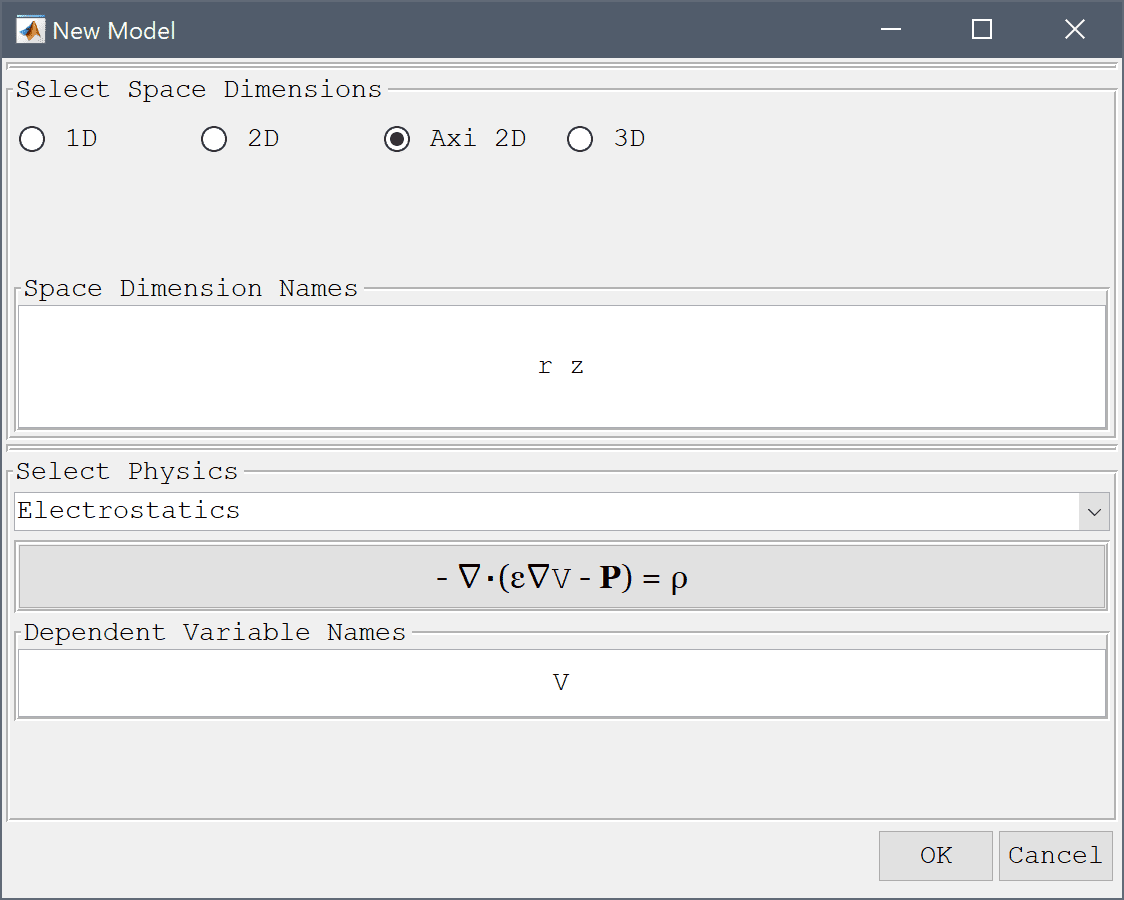4. Press OK to finish the physics mode selection.

Start by creating three circles all centered at (0, 0) with radius 0.003, 0.01, and 0.012, respectively.

1. Select Circle from the Geometry menu.
2. Enter 0.003 into the radius edit field.
3. Press OK to finish and close the dialog box.
4. Select Circle from the Geometry menu.
5. Enter 0.01 into the radius edit field.
6. Press OK to finish and close the dialog box.
7. Select Circle from the Geometry menu.
8. Enter 0.012 into the radius edit field.
9. Press OK to finish and close the dialog box.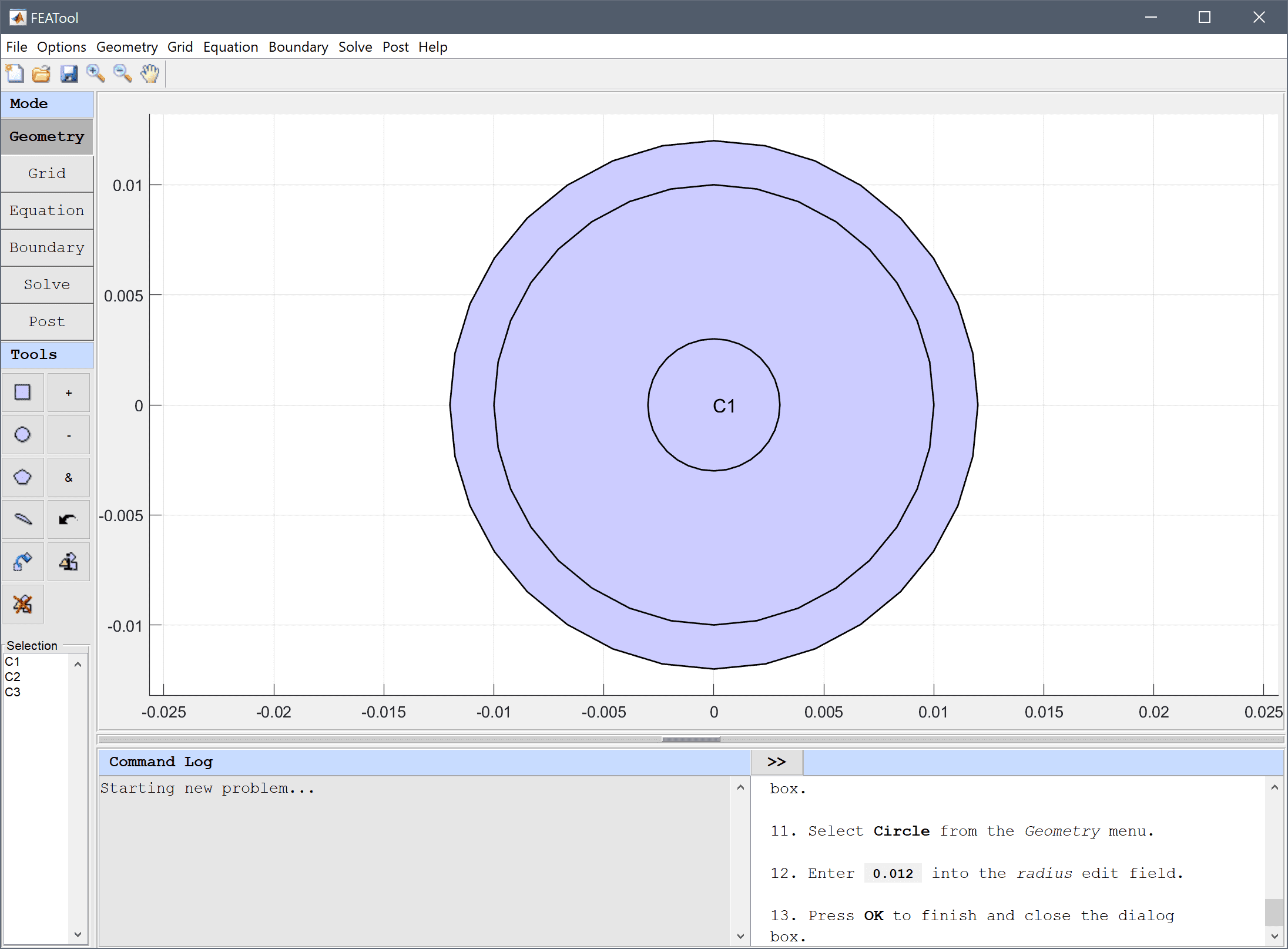Create three rectangles on the left side of the symmetry axis (r<0) so that they cover the left side of the circles (extending a somewhat above, below and to the left).

1. Select Rectangle from the Geometry menu.
2. Enter -0.013 into the xmin edit field.
3. Enter 0 into the xmax edit field.
4. Enter -0.013 into the ymin edit field.
5. Enter 0.013 into the ymax edit field.
6. Press OK to finish and close the dialog box.
7. Select R1 in the geometry object Selection list box.
8. Press the Copy and/or transform selected geometry object Toolbar button.
9. Select the Make copy of geometry object check box.
10. Press OK to finish and close the dialog box.
11. Select R2 in the geometry object Selection list box.
12. Press the Copy and/or transform selected geometry object Toolbar button.
13. Select the Make copy of geometry object check box.
14. Press OK to finish and close the dialog box.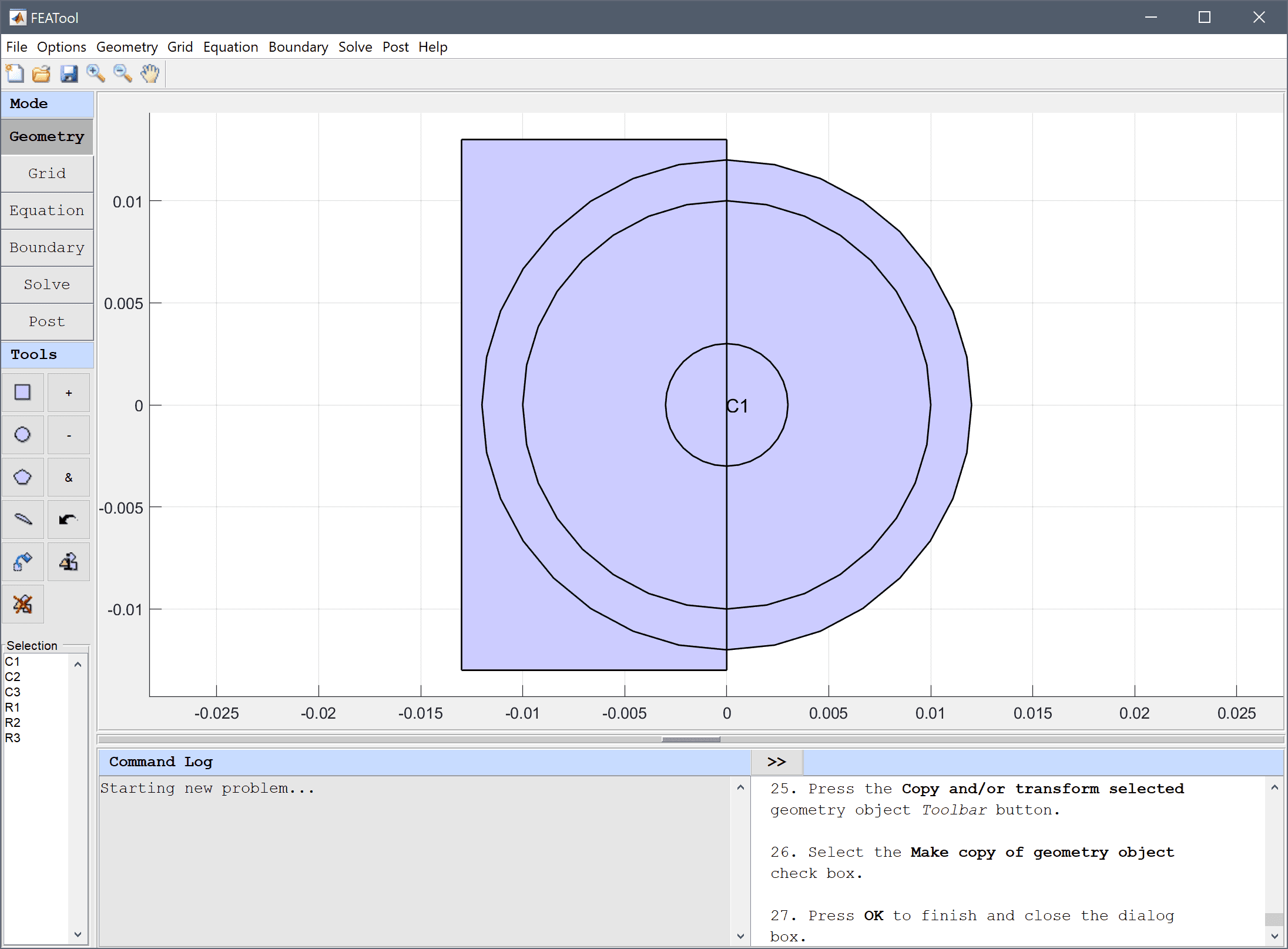One by one subtract the rectangles from the corresponding circles so that only half circles on right side half plane remain.

1. Select Combine Objects... from the Geometry menu.
2. Enter C1 - R1 into the Geometry Formula edit field.
3. Press OK to finish and close the dialog box.
4. Select C2 and R2 in the geometry object Selection list box.
5. Press the - / Subtract geometry objects Toolbar button.
6. Select C3 and R3 in the geometry object Selection list box.
7. Press the - / Subtract geometry objects Toolbar button.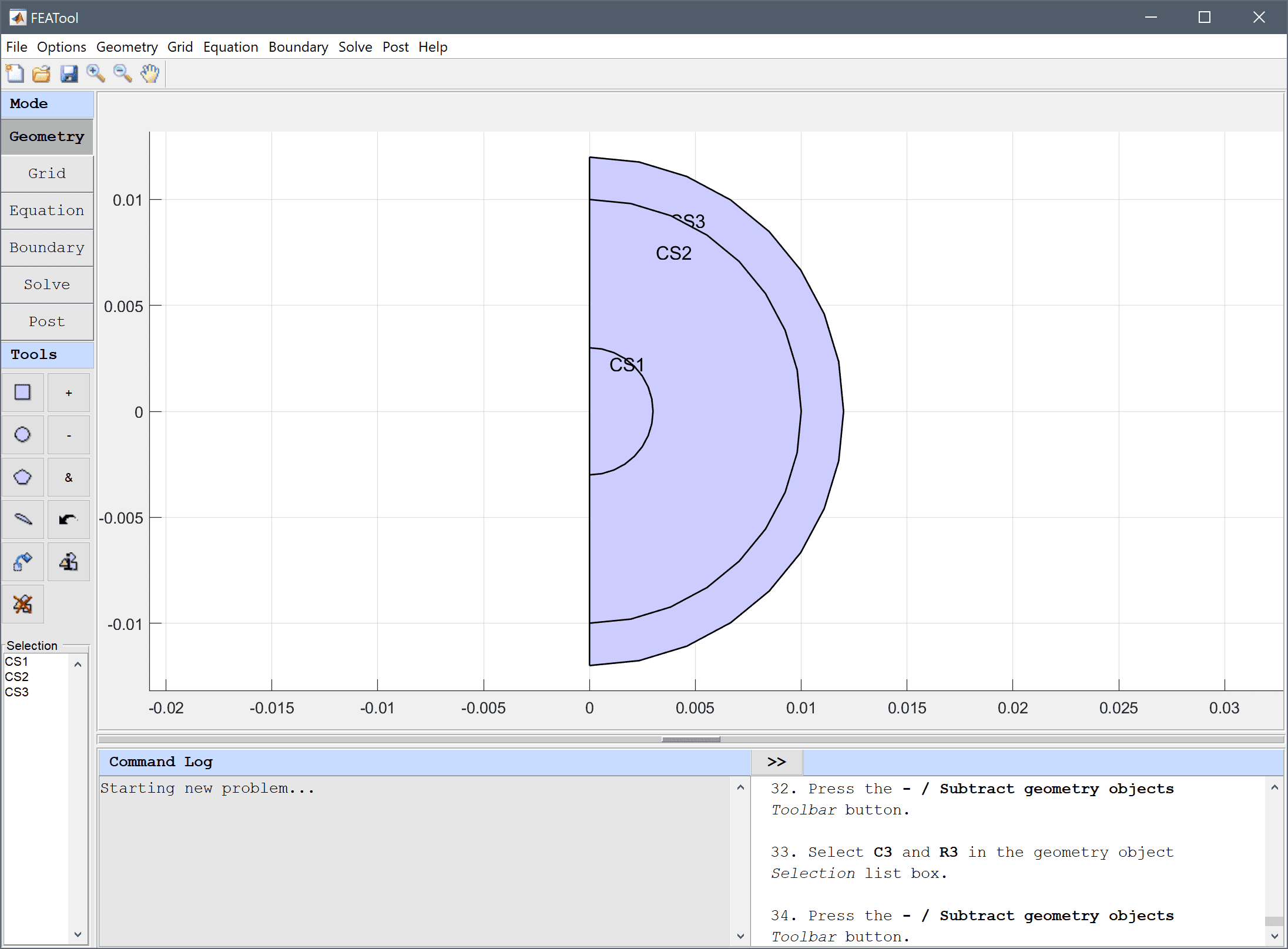8. Switch to Grid mode by clicking on the corresponding Mode Toolbar button.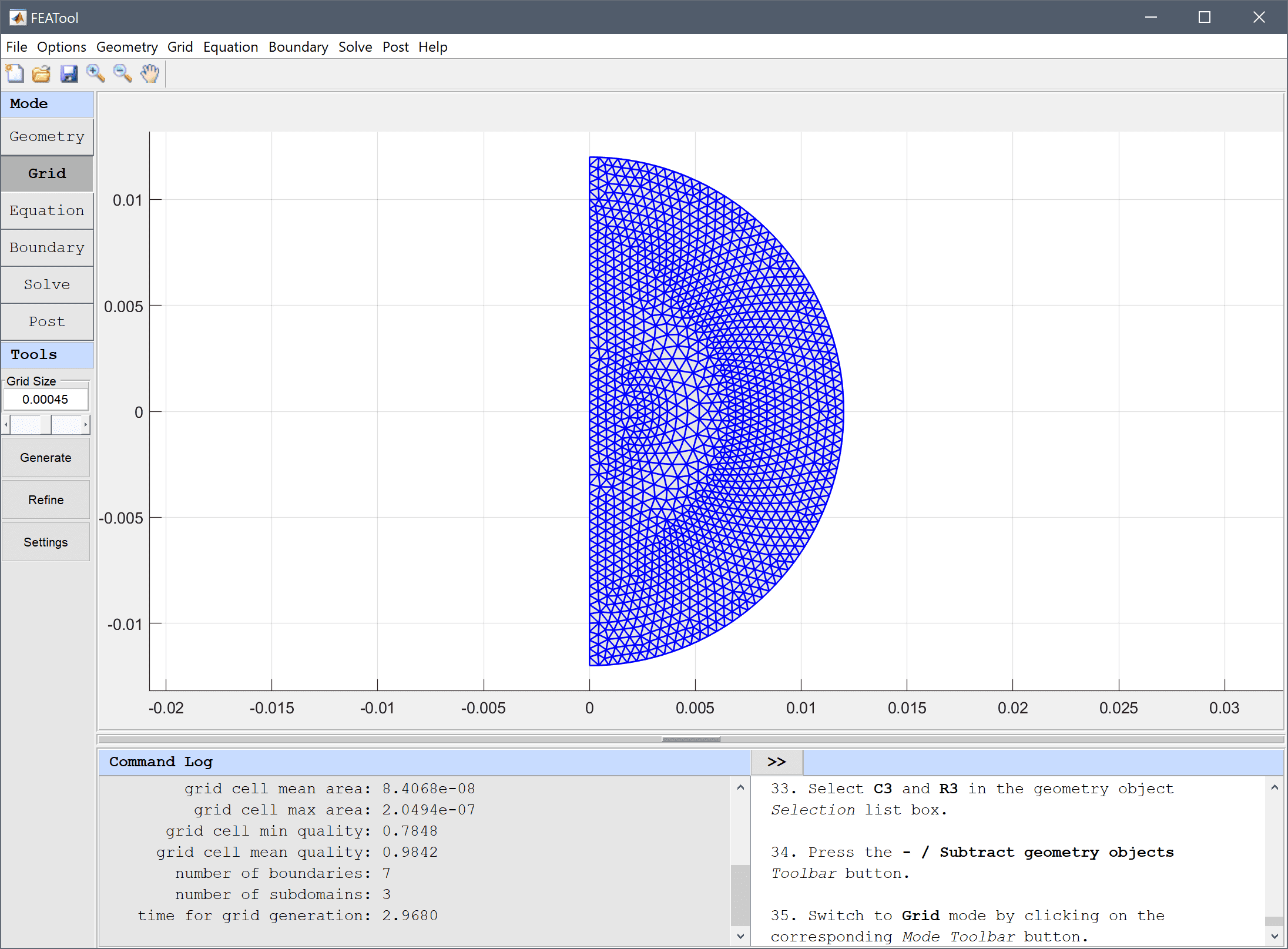9. Press the Equation mode button to switch from grid mode to physics and equation/subdomain specification mode. In the Equation Settings dialog box that automatically opens, select all Subdomains (1-3) and enter sigma+epsr*eps0/tscale for the Permittivity, ε, and rho/tscale for the Space charge density, ρ.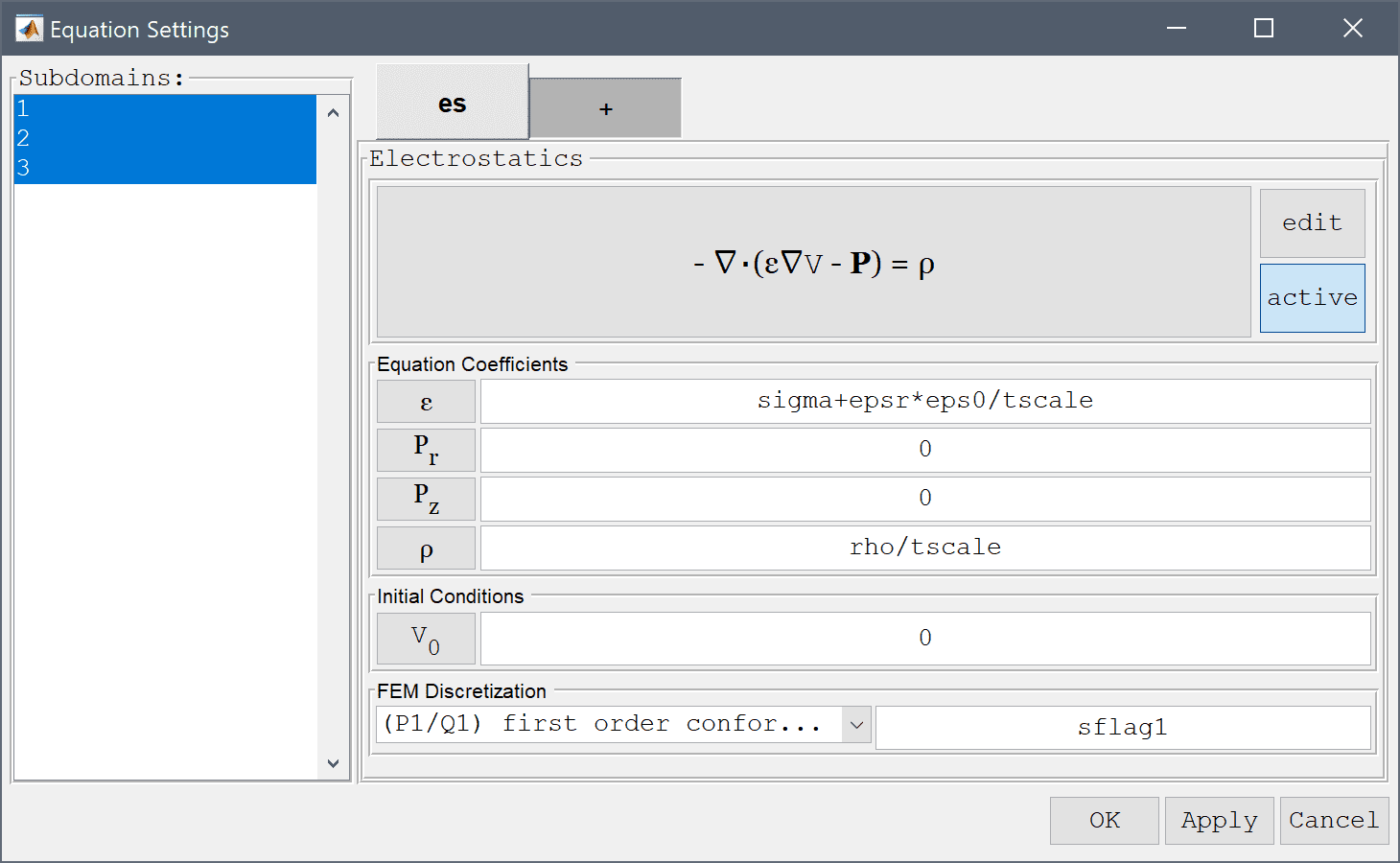10. Press the Constants Toolbar button, or select the corresponding entry from the Equation menu, and enter the following variables for the capacitor parameters in the Model Constants and Expressions dialog box. Press Enter after the last expression or use the Add Row button to expand the expression list. Also note that values for different subdomains are entered a a space separated list.
Name Expression
r1 0.003
r2 0.01
r3 0.012
sigma 0   6e7   6e7
eps0 8.85e-12
epsr 3.9   1   1
tscale 1e-17
rho 0   q0*3/4/pi/(r1^3)   -q0*3/4/pi/(r3^3-r2^3)
q0 6e-11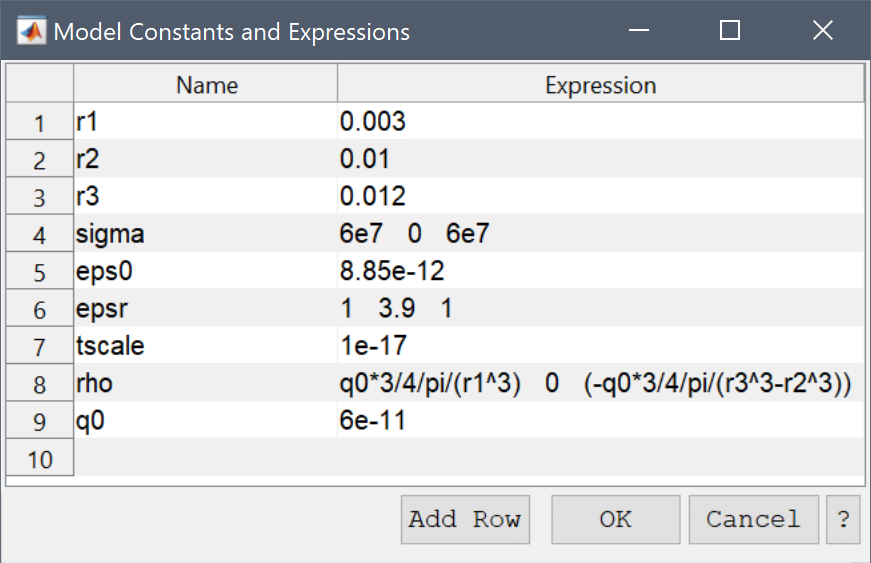1. Switch to Boundary mode by clicking on the corresponding Mode Toolbar button.
2. Select 1, 2, 3, 6, and 7 in the Boundaries list box.
3. Select Insulation/symmetry from the Electrostatics drop-down menu.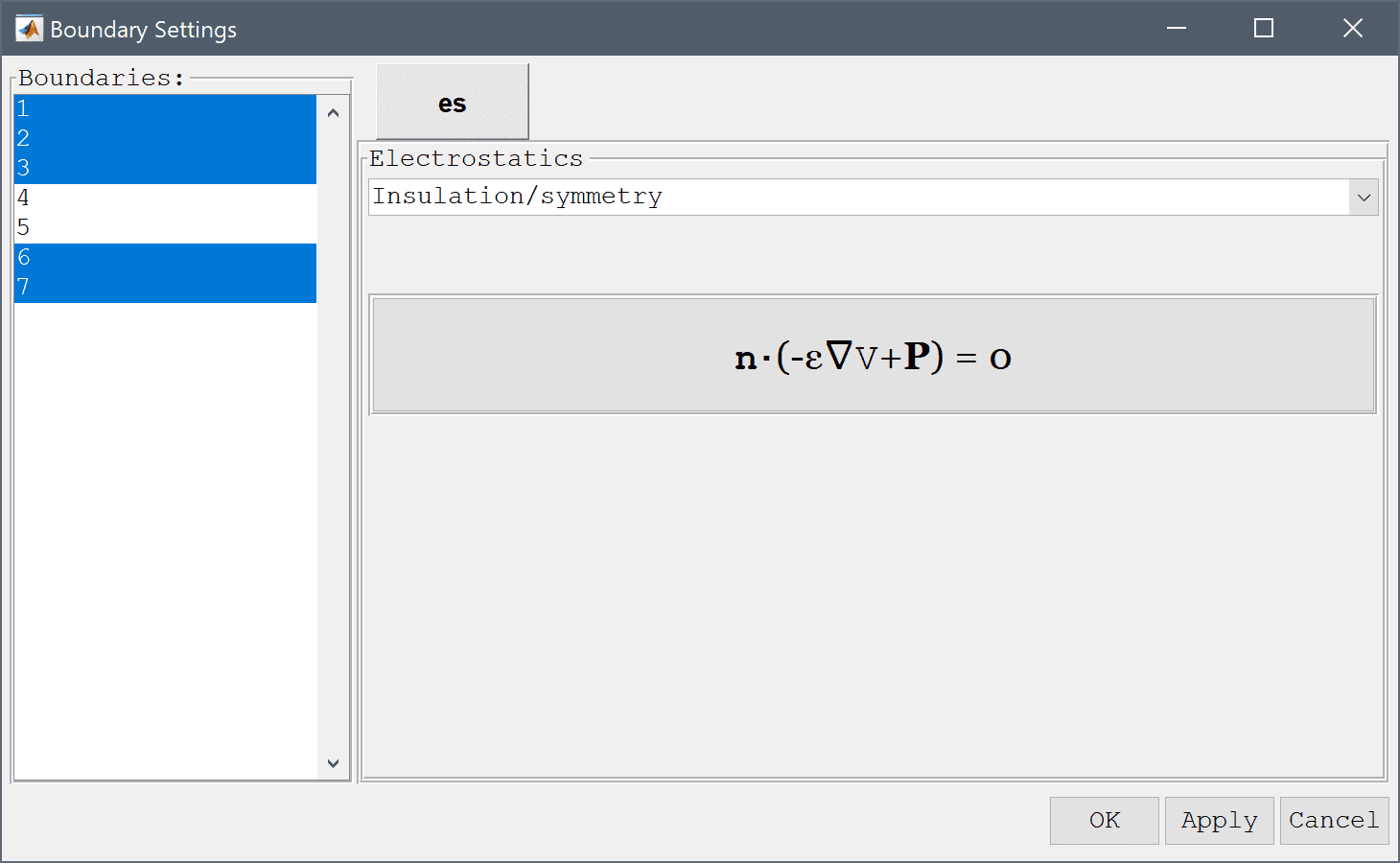4. Select 4 and 5 in the Boundaries list box.
5. Select Ground/antisymmetry from the Electrostatics drop-down menu.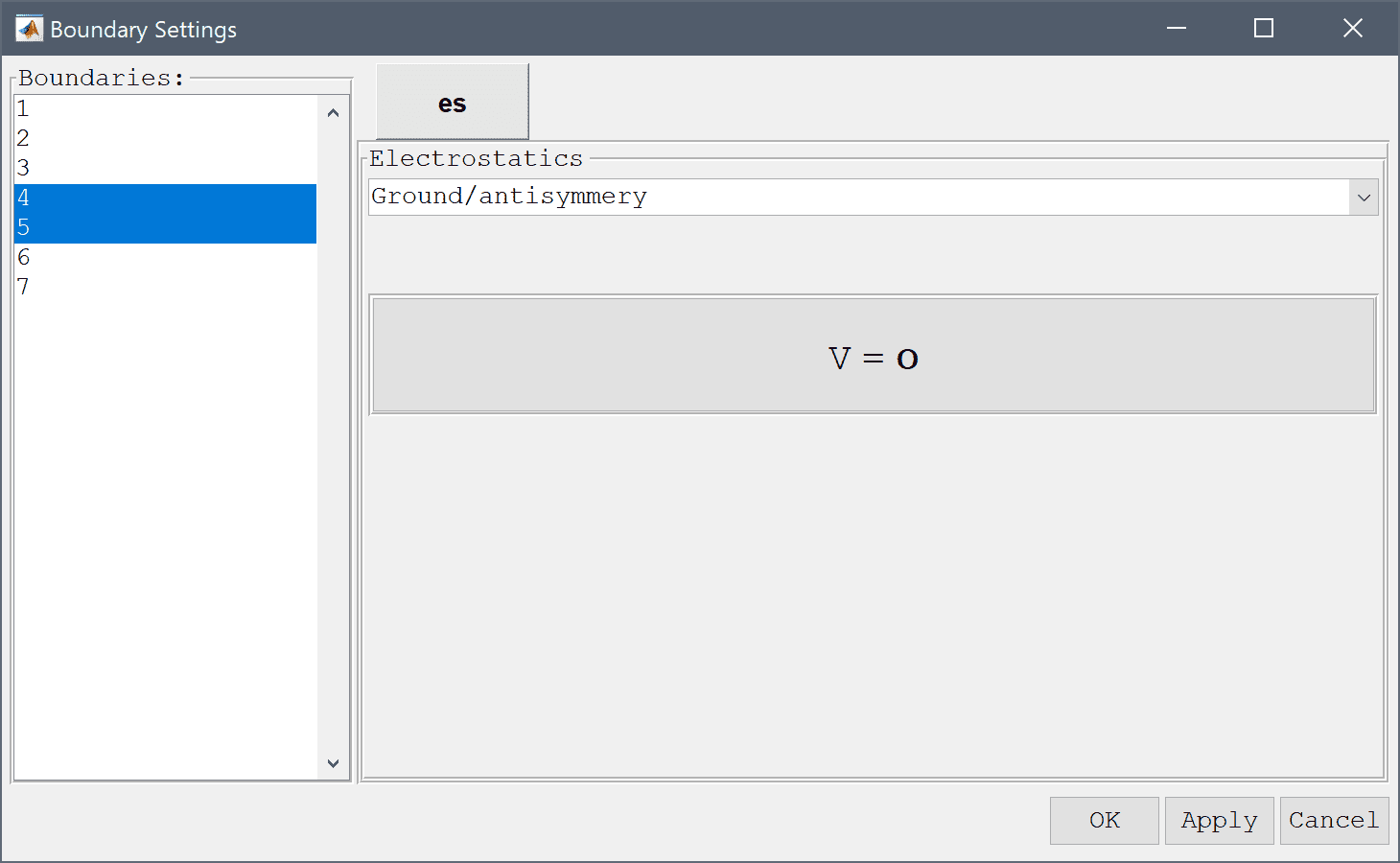6. Press OK to finish the boundary condition specification.
7. Switch to Solve mode by clicking on the corresponding Mode Toolbar button, and press the = Toolbar button to call the solver. After the problem has been solved, FEATool automatically changes to Postprocessing mode and plots the electric potential V.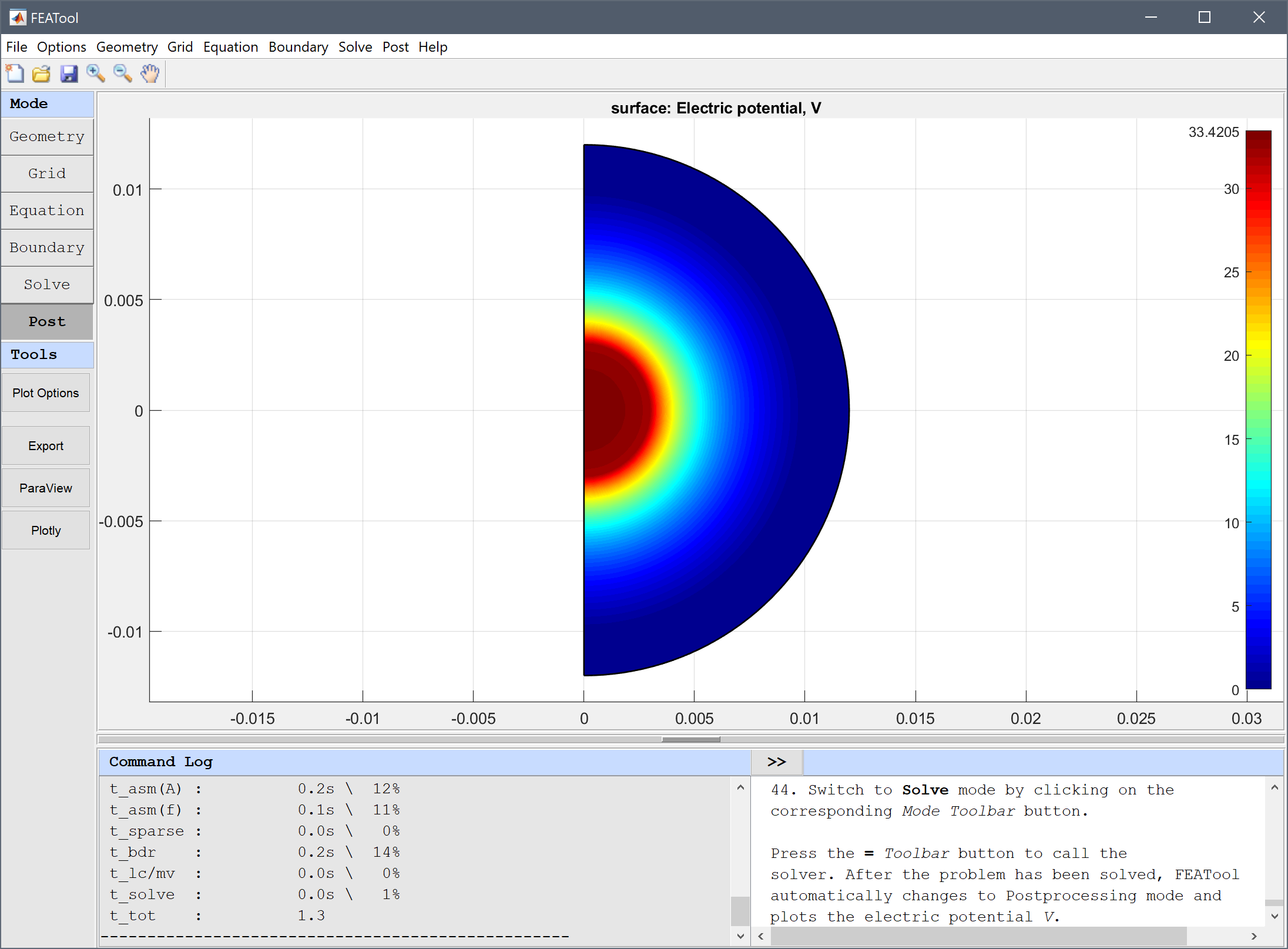8. Open the Postprocessing Settings dialog box and plot the current density which can be expressed as sigma*sqrt(Vr^2+Vz^2)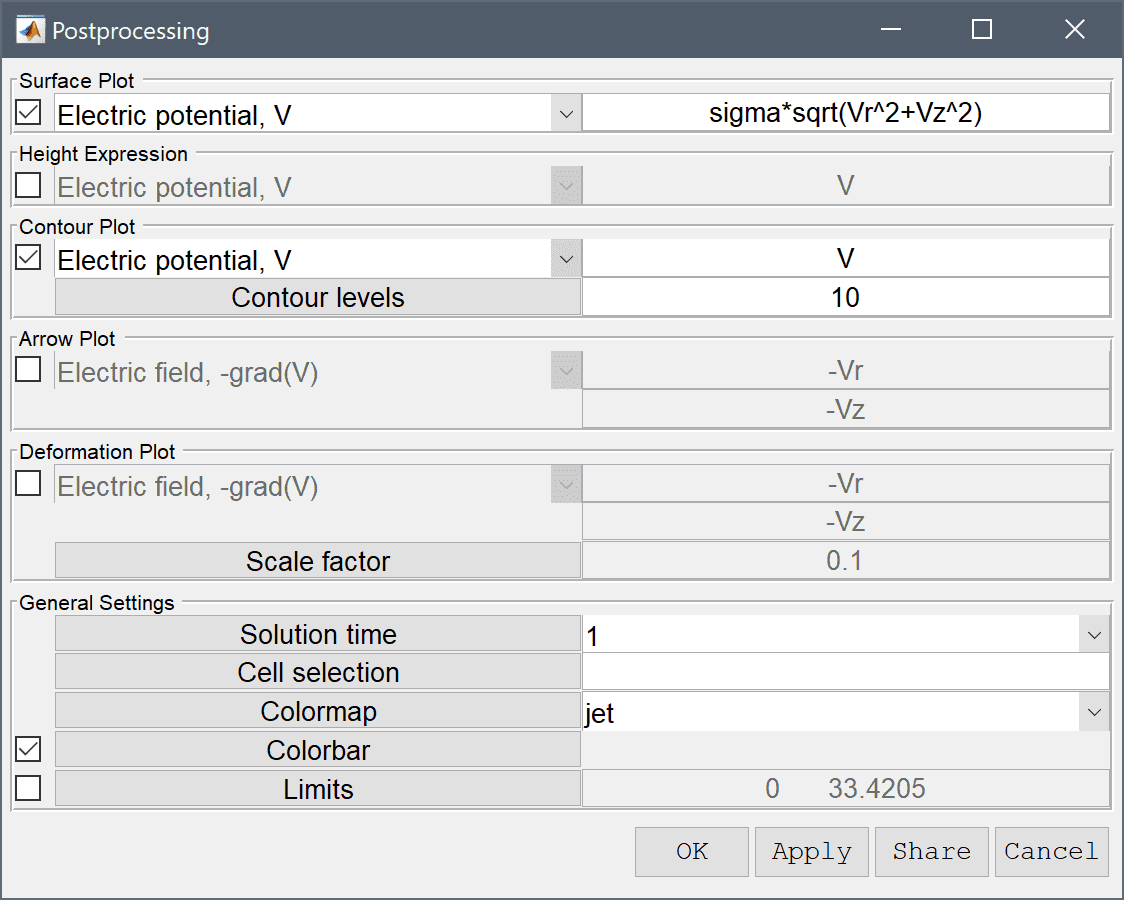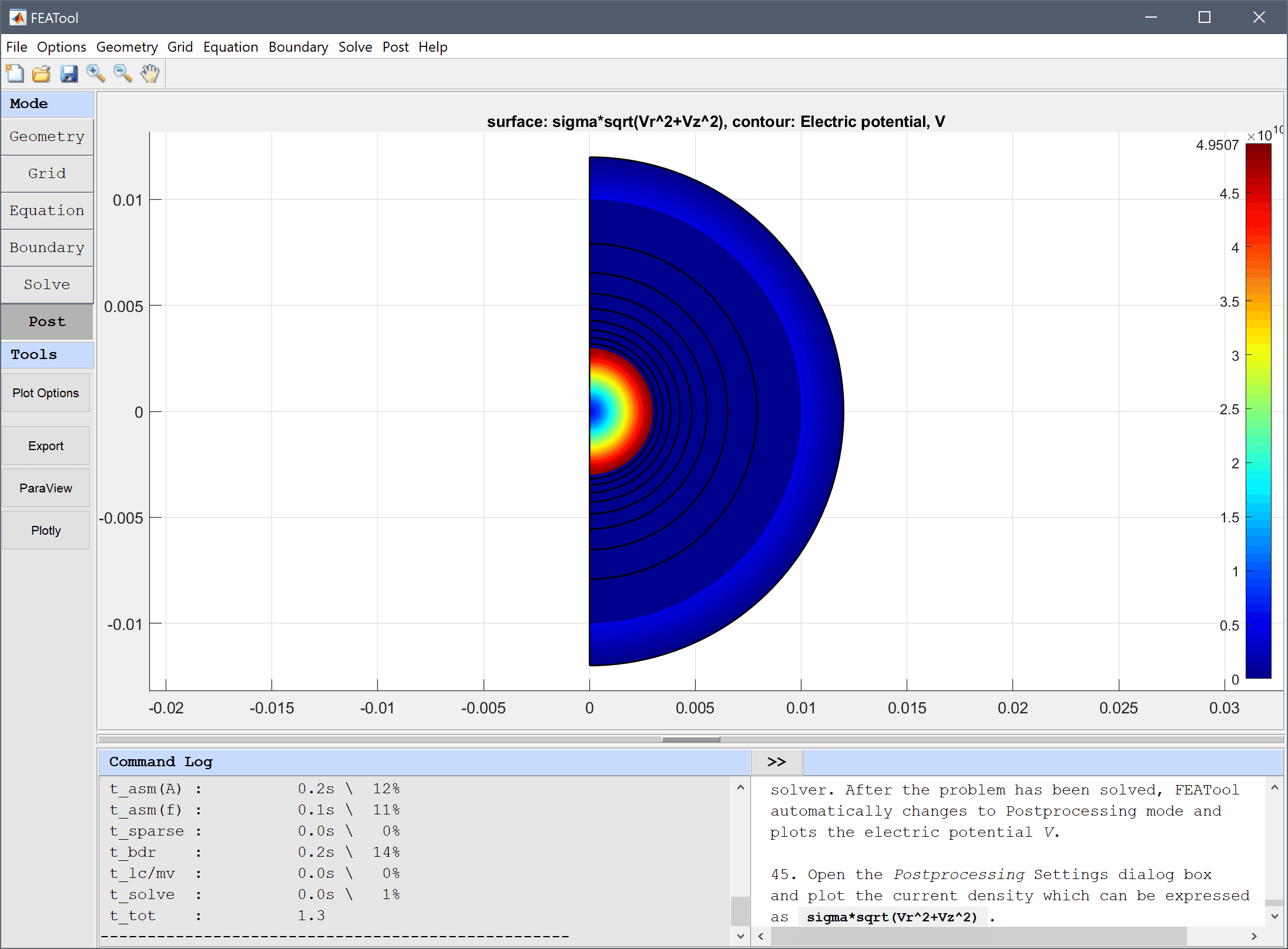To verify the solution, one can calculate the capacitance as q0/( Vmax - Vmin ) where Vmax can be found by using the Min/Max Evaluation... option from the Post menu (Vmin will be 0).

1. Select Min/Max Evaluation... from the Post menu.
2. Enter q0/V into the edit field.
3. Press the Apply button.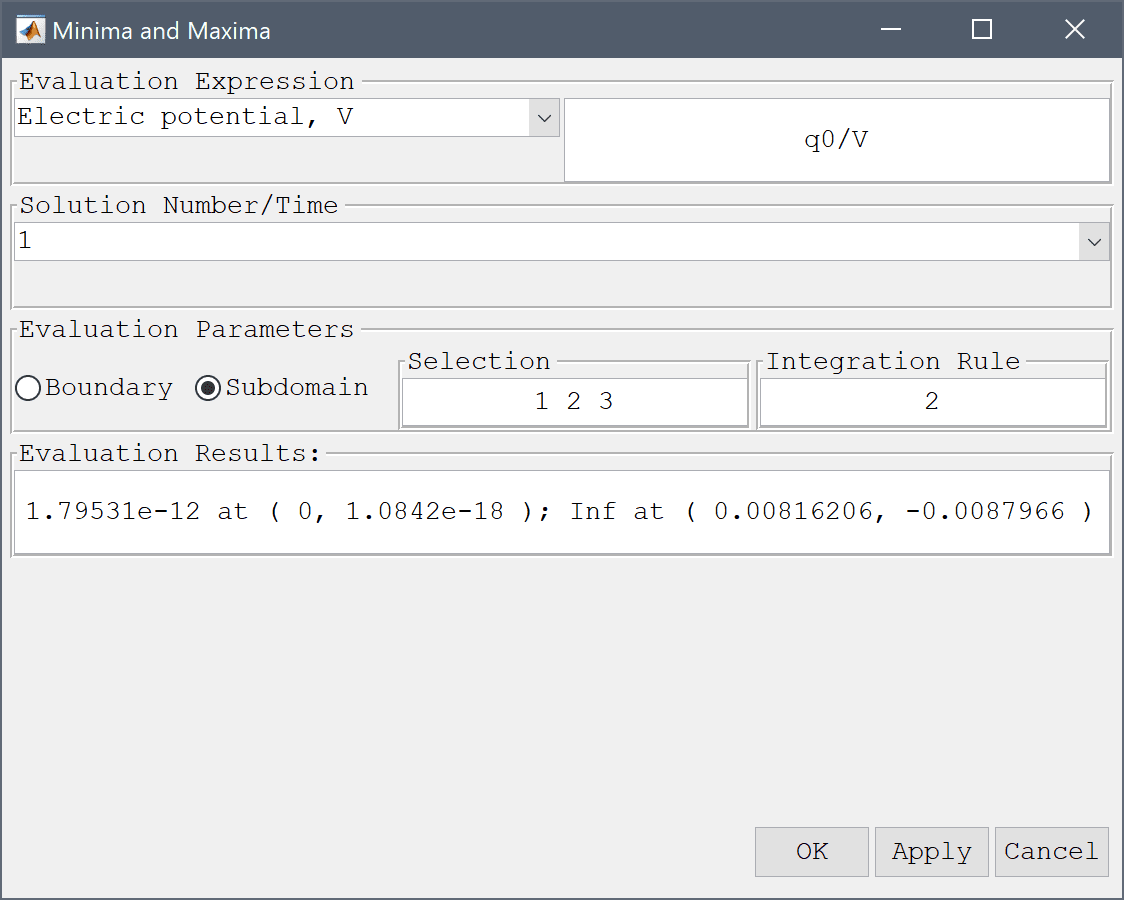Alternatively, one can integrate the expression for the energy E = ε0εr(Vr2+Vz2)πr over all subdomains, where the capacitance then can be calculated as q02/(2·E).

Compare the computed capacitances with the analytical expression 4*πεrε0/( 1/0.003 - 1/0.01 ) which in this example should be equal to 1.8588e-12.

The spherical capacitor electromagnetics model has now been completed and can be saved as a binary (.fea) model file, or exported as a programmable MATLAB m-script text file (available as the example ex_electrostatics2 script file), or GUI script (.fes) file.

# CLI Postprocessing

To visualize the full 3D solution from the axisymmetic model, the data can be exported and processed on the MATLAB command line interface (CLI) console with the Export Model Data Struct to MATLAB option from the File menu. The postrevolve and postplot functions can then be applied to revolve and visualize the data, for example

fea_revolved = postrevolve( fea, 24, 0.75 );

postplot( fea_revolved, 'surfexpr', 'V', ...
'parent', figure, 'axis', 'off', 'colorbar', 'off' )
view(70, 25)